ForEach Controller in Jmeter iterates through an array of variables.

In this JMeter tutorial, we’ll use the ForEach Controller to loop through a JSON Array.

There are times when we need to parse a response and extract certain information from it. For example, when testing an API, we could get a JSON response which could contain JSON Arrays.

Then, we need to loop through the array and for each element perform an action. In JMeter, we can use the ForEach Controller to iterate through the JSON Array.

## How to Use JMeter ForEach Controller

In this example, we will be making a GET request to a resource which returns a JSON response.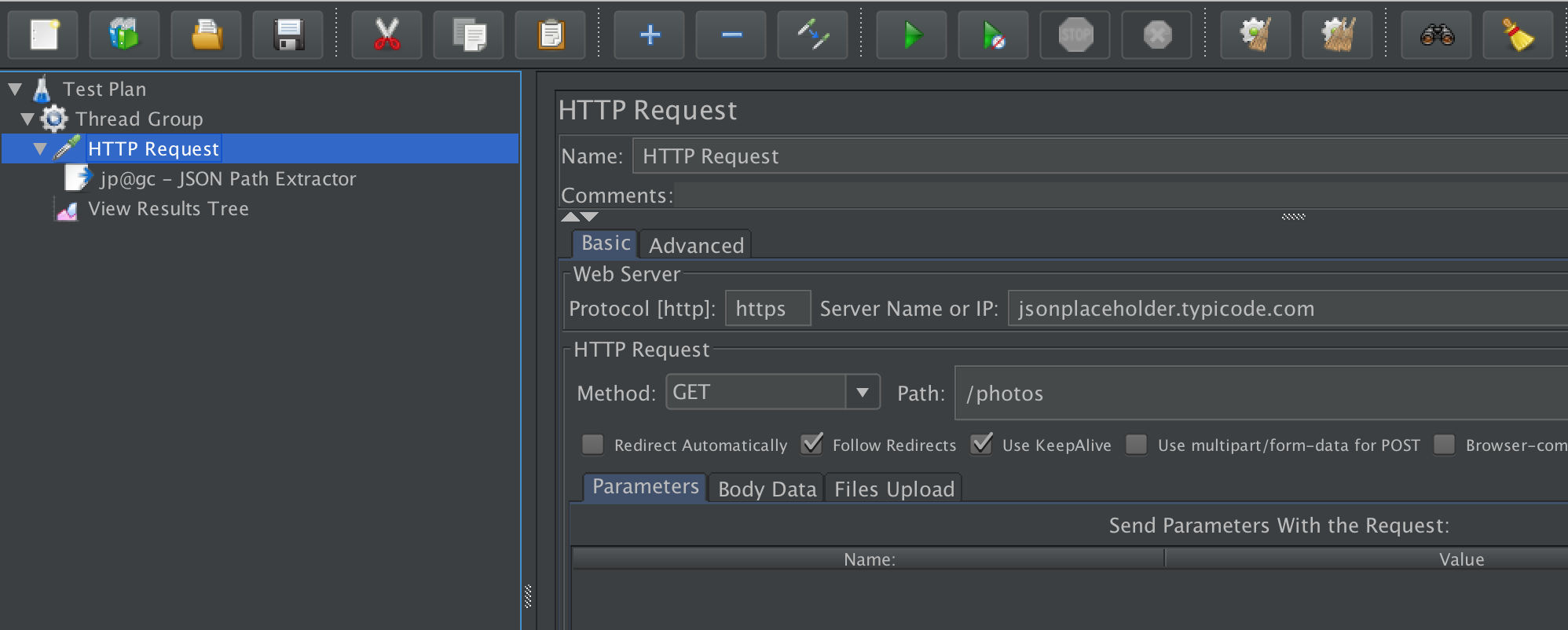The response contains an Array of JSON objects.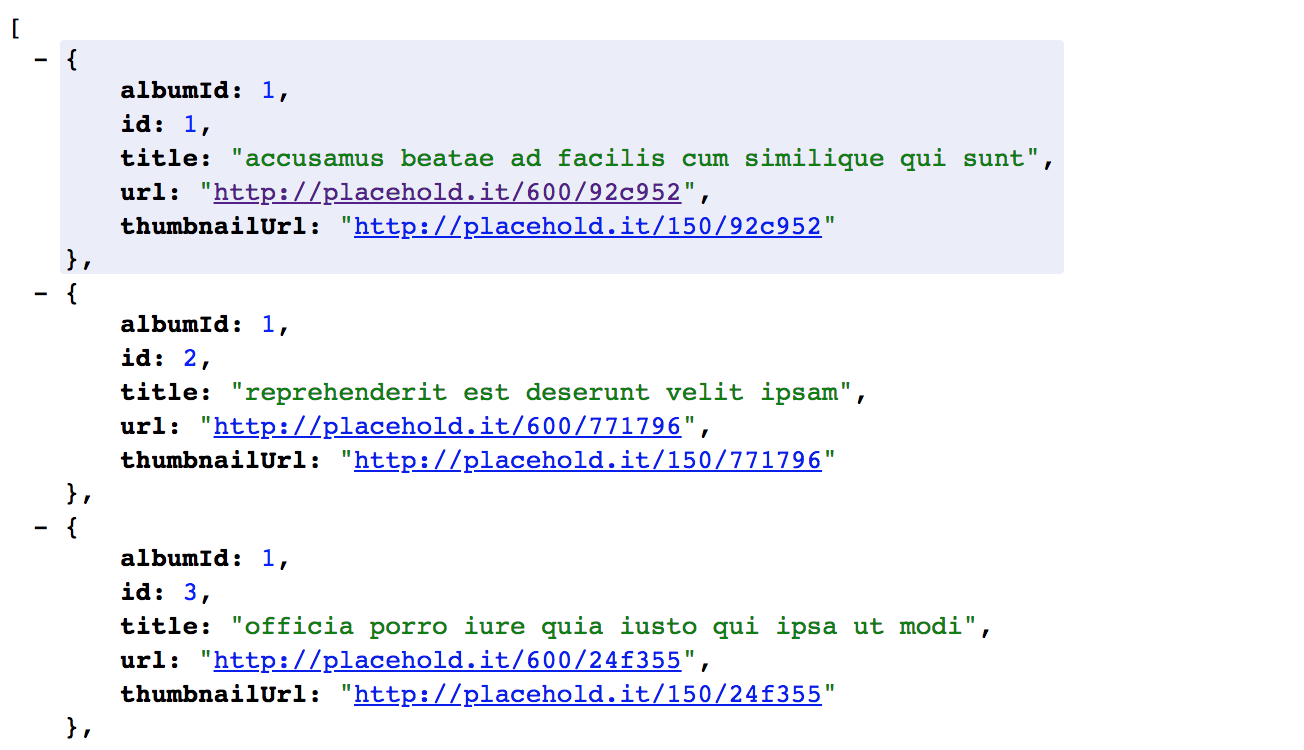For each object, we need to extract the URL which we can do via JSONPath.

The JSONPath to get all the URL’s in the above response is $.[*].url. Once we parse the JSON response and extract the URLs, we then have an array of Strings, basically the URLs. We save this array in a variable called url_array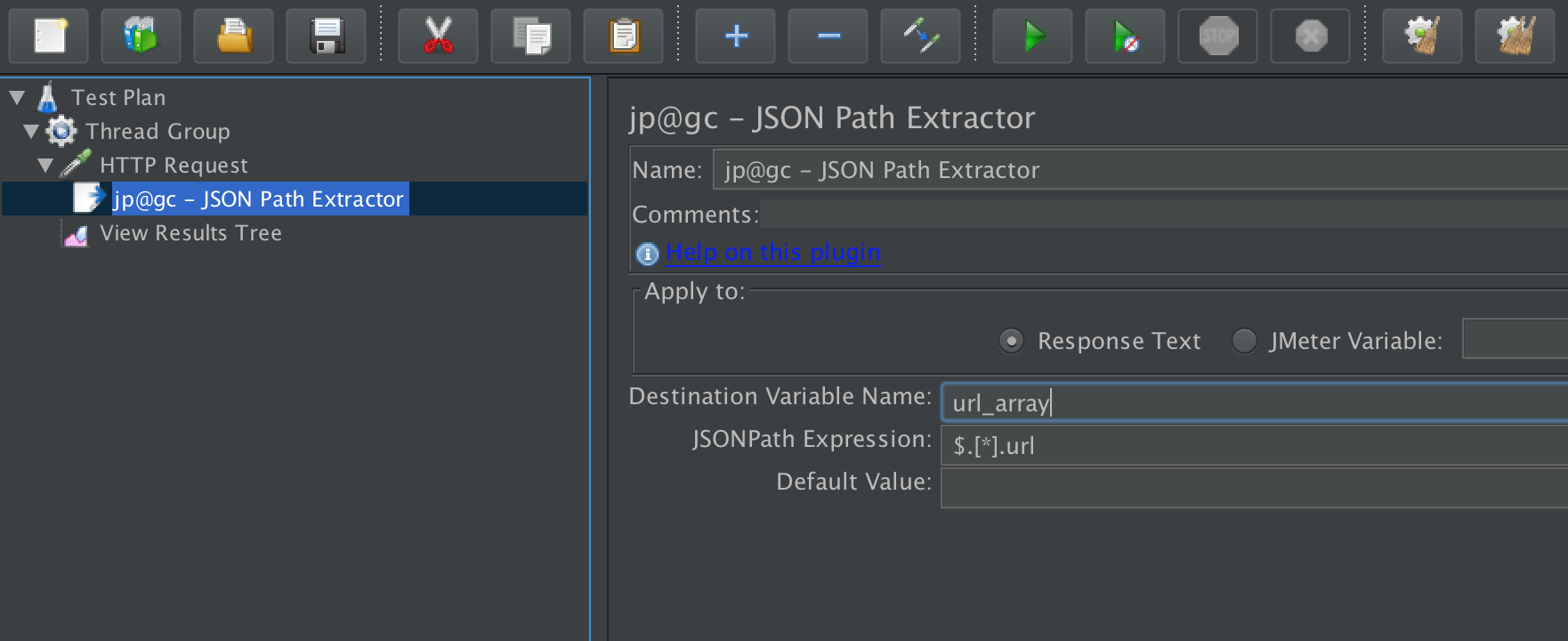Now suppose that for each element of the String array, we want to make a request to the URL. In JMeter, this is done by using the ForEach Controller. To add the ForEach Controller to your test plan, right click on Thread Group > Add > Logic Controller > ForEach Controller The ForEach Controller requires two parameters: • Input variable prefix • Output variable name The Input variable prefix takes the name of the array variable, in this example, url_array . For the Output variable name, we will assign a variable, in this example, url_index which we’re going to use in the subsequent request.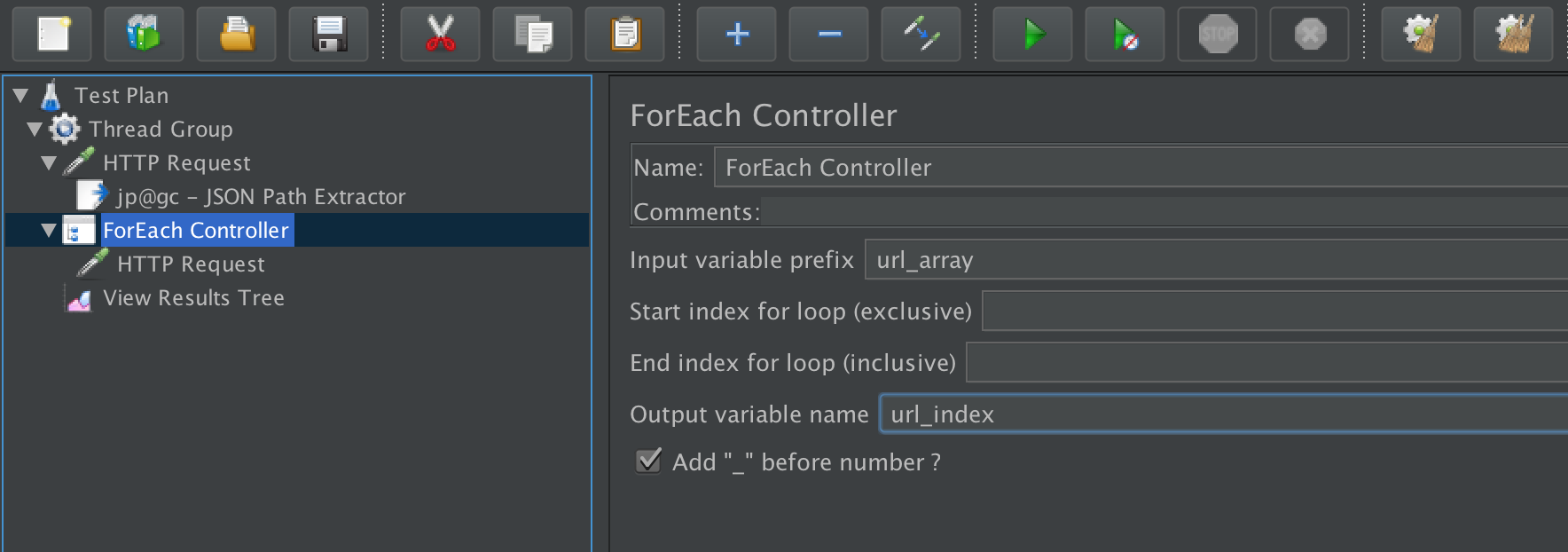Then, in our subsequent requests, we can extract each value by using ${url_index}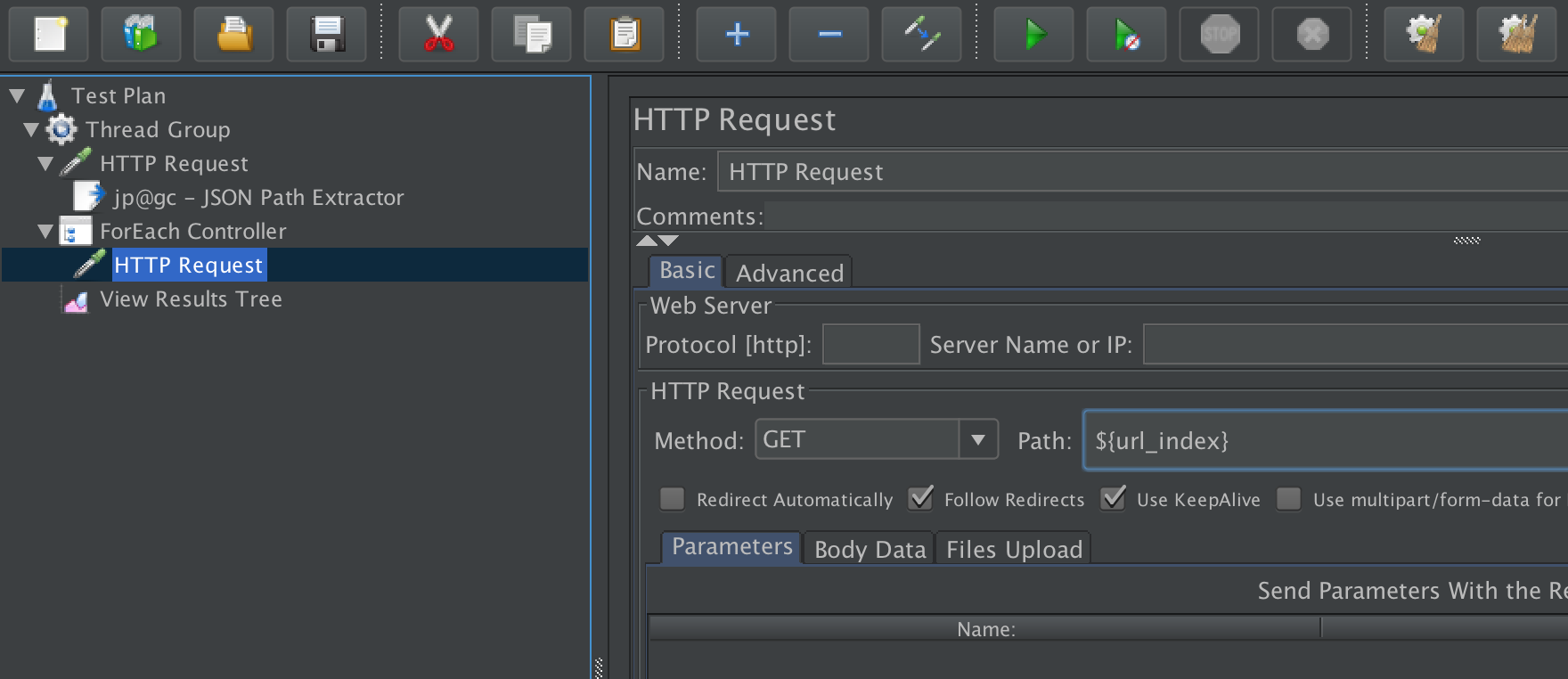This will now loop through each entry in the JSON Array and make the HTTP requests to the URLs.